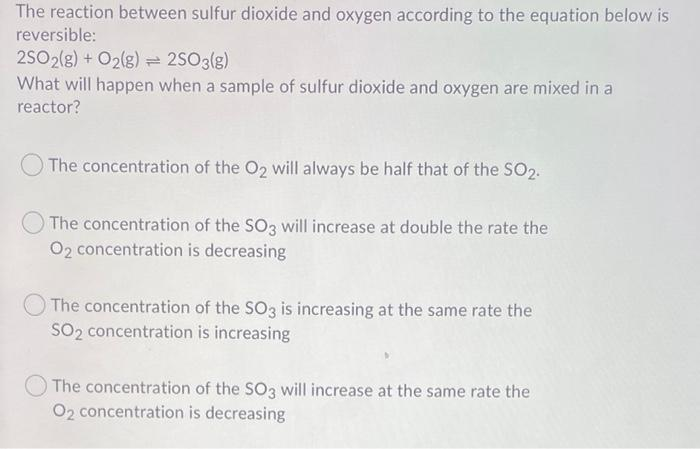# Question Solved1 AnswerThe reaction between sulfur dioxide and oxygen according to the equation below is reversible: 2SO2(g) + O2(g) = 2503(g) What will happen when a sample of sulfur dioxide and oxygen are mixed in a reactor? The concentration of the O2 will always be half that of the SO2. The concentration of the SO3 will increase at double the rate the O2 concentration is decreasing The concentration of the SO3 is increasing at the same rate the SO2 concentration is increasing The concentration of the SO3 will increase at the same rate the O2 concentration is decreasingTranscribed Image Text: The reaction between sulfur dioxide and oxygen according to the equation below is reversible: 2SO2(g) + O2(g) = 2503(g) What will happen when a sample of sulfur dioxide and oxygen are mixed in a reactor? The concentration of the O2 will always be half that of the SO2. The concentration of the SO3 will increase at double the rate the O2 concentration is decreasing The concentration of the SO3 is increasing at the same rate the SO2 concentration is increasing The concentration of the SO3 will increase at the same rate the O2 concentration is decreasing
More
Transcribed Image Text: The reaction between sulfur dioxide and oxygen according to the equation below is reversible: 2SO2(g) + O2(g) = 2503(g) What will happen when a sample of sulfur dioxide and oxygen are mixed in a reactor? The concentration of the O2 will always be half that of the SO2. The concentration of the SO3 will increase at double the rate the O2 concentration is decreasing The concentration of the SO3 is increasing at the same rate the SO2 concentration is increasing The concentration of the SO3 will increase at the same rate the O2 concentration is decreasing